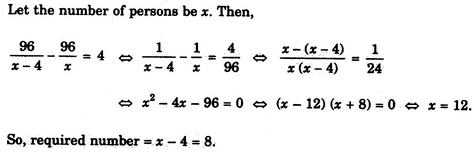# Verbal Reasoning - Arithmetic Reasoning

## Why Verbal Reasoning Arithmetic Reasoning?

In this section you can learn and practice Verbal Reasoning Questions based on "Arithmetic Reasoning" and improve your skills in order to face the interview, competitive examination and various entrance test (CAT, GATE, GRE, MAT, Bank Exam, Railway Exam etc.) with full confidence.

## Where can I get Verbal Reasoning Arithmetic Reasoning questions and answers with explanation?

IndiaBIX provides you lots of fully solved Verbal Reasoning (Arithmetic Reasoning) questions and answers with Explanation. Solved examples with detailed answer description, explanation are given and it would be easy to understand. All students, freshers can download Verbal Reasoning Arithmetic Reasoning quiz questions with answers as PDF files and eBooks.

## Where can I get Verbal Reasoning Arithmetic Reasoning Interview Questions and Answers (objective type, multiple choice)?

Here you can find objective type Verbal Reasoning Arithmetic Reasoning questions and answers for interview and entrance examination. Multiple choice and true or false type questions are also provided.

## How to solve Verbal Reasoning Arithmetic Reasoning problems?

You can easily solve all kind of Verbal Reasoning questions based on Arithmetic Reasoning by practicing the objective type exercises given below, also get shortcut methods to solve Verbal Reasoning Arithmetic Reasoning problems.

### Exercise :: Arithmetic Reasoning - Section 1

1.

The total of the ages of Amar, Akbar and Anthony is 80 years. What was the total of their ages three years ago ?

 A. 71 years B. 72 years C. 74 years D. 77 years

Explanation:

Required sum = (80 - 3 x 3) years = (80 - 9) years = 71 years.

2.

Two bus tickets from city A to B and three tickets from city A to C cost Rs. 77 but three tickets from city A to B and two tickets from city A to C cost Rs. 73. What are the fares for cities B and C from A ?

 A. Rs. 4, Rs. 23 B. Rs. 13, Rs. 17 C. Rs. 15, Rs. 14 D. Rs. 17, Rs. 13

Explanation:

Let Rs. x be the fare of city B from city A and Rs. y be the fare of city C from city A.

Then, 2x + 3y = 77 ...(i) and

3x + 2y = 73 ...(ii)

Multiplying (i) by 3 and (ii) by 2 and subtracting, we get: 5y = 85 or y = 17.

Putting y = 17 in (i), we get: x = 13.

3.

An institute organised a fete and 1/5 of the girls and 1/8 of the boys participated in the same. What fraction of the total number of students took part in the fete ?

 A. 2/13 B. 13/40 C. Data inadequate D. None of these

Explanation:

No answer description available for this question. Let us discuss.

4.

A number of friends decided to go on a picnic and planned to spend Rs. 96 on eatables. Four of them, however, did not turn up. As a consequence, the remaining ones had to contribute Rs. 4 each extra. The number of those who attended the picnic was

 A. 8 B. 12 C. 16 D. 24

Explanation:5.

A, B, C, D and E play a game of cards. A says to B, "If you give me three cards, you will have as many as E has and if I give you three cards, you will have as many as D has." A and B together have 10 cards more than what D and E together have. If B has two cards more than what C has and the total number of cards be 133, how many cards does B have ?

 A. 22 B. 23 C. 25 D. 35

Explanation:

Clearly, we have :

B-3 = E ...(i)

B + 3 = D ...(ii)

A+B = D + E+10 ...(iii)

B = C + 2 ...(iv)

A+B + C + D + E= 133 ...(v)

From (i) and (ii), we have : 2 B = D + E ...(vi)

From (iii) and (vi), we have : A = B + 10 ...(vii)

Using (iv), (vi) and (vii) in (v), we get:

(B + 10) + B + (B - 2) + 2B = 1335B = 125B = 25.